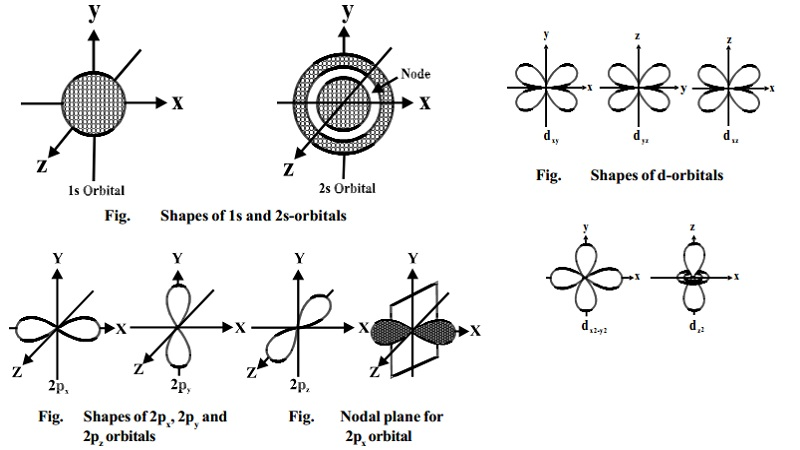Home | | Chemistry | | Chemistry | Shapes of orbitals: s, p, d Shapes

# Shapes of orbitals: s, p, d ShapesAn orbital is the region of space around the nucleus within which the probability of finding an electron of given energy is maximum .The shape of this region (electron cloud) gives the shape of the orbital. The plot of angular wave functions or square of angular wave functions (probability functions) give us the shapes of orbitals.These two plots differ only slightly. Let us consider the individual shapes.

Shapes of orbitals

An orbital is the region of space around the nucleus within which the probability of finding an electron of given energy is maximum .The shape of this region (electron cloud) gives the shape of the orbital. The plot of angular wave functions or square of angular wave functions (probability functions) give us the shapes of orbitals.These two plots differ only slightly. Let us consider the individual shapes.

Shape of s-orbitals

For s-orbitals, when l = 0, the value of m is 0 i.e., there is only one possible orientation. This means that the probability of finding an electron is the same in all directions at a given distance from the nucleus. It should, therefore, be spherical in shape. Hence all s- orbitals are non- directional and spherically symmetrical about the nucleus.The size of an s-orbital depends upon value of the principal quantum number n. Greater the value of 'n' larger is the size of the orbital.

An important feature of the 2s-orbital is that there is a spherical shell within this orbital where the probability of finding the electron is zero (nearly). This is called a node or nodal surface. In 2s orbital there is one spherical node. The number of nodal surfaces or nodes in s-orbital of any energy level is equal to (n-1), where n is the principal quantum number.

Shape of p-orbitals

For p-subshell l = 1, there are three values of m namely -1, 0, +1. It means that p orbitals can have three possible orientations. These three p-orbitals are equal in energy (degenerate state) but differ in their orientations. Each p-orbital consists of two lobes symmetrical about a particular axis. Depending upon the orientation of the lobes, these are denoted as 2px , 2py and 2pz accordingly as they are symmetrical about X,Y and Z - axis respectively.The lines in the figure represents the cross-section of the three dimensional boundary surface of p-orbitals. The boundary surface means the surface which encloses 90 percent of the dots representing the electrons. Two lobes of each p-orbital are separated by a nodal plane (a plane having zero electron density). For example, for 2px orbital, YZ plane is the nodal plane x

Thus, p-orbitals have dumb-bell shape and have directional character. The probability of finding the electron is equal in both the lobes. The p-orbitals of higher energy levels have similar shapes although their size are bigger.

Shape of d-orbitals

For d-subshell, l = 2, there are five values of m namely -2, -1, 0, 1, 2. It

means d- orbitals can have five orientations. These are represented by dxy, dyz,dzx, dx2-y2 and dz2; for example, 3dxy, 3dyz, 3dzx, 3dx2-y2 and 3dz2. The dxy, dyz and dzx orbitals have same shape i.e., clover leaf shape but they lie in XY, YZ and ZX-

planes respectively.The dz2 orbital is symmetrical about Z-axis and has a dumb - bell shape with a doughnut shaped electron cloud in the centre. The dx2-y2 orbital is also clovar leaf shaped but its leaves are directed along the X and Y- axis.

The reason for the presence of four lobes in any nd orbital lies in the fact that the d - orbitals have two nodes, and hence two changes in algebraic sign of ψ, which lead to four lobes.

Study Material, Lecturing Notes, Assignment, Reference, Wiki description explanation, brief detail

Related Topics

Copyright © 2018-2021 BrainKart.com; All Rights Reserved. (BS) Developed by Therithal info, Chennai.